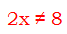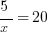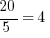# Common GMAT Math Mistakes: What To Avoid

All of us are susceptible to make common mistakes, so of course we are also likely to make some of the most common GMAT math mistakes. We’ve written a lot about developing critical thinking skills and good study habits, but we are going to always make mistakes.

One of my favorite GMAT bloggers, Mike McGarry with Magoosh, has written a post about the most common GMAT math mistakes. How many of these have you found yourself making?

#1: Dropping the Negative Sign

Suppose you are solving the equation

5 – 2x = 13

We want to isolate x.  One tactic would be to begin by subtracting 5 from both sides.  On the right, 13 – 5 = 8.  On the left, the 5′s cancel, but with what are we left?  It would be a mistake to subtract 5 and wind up with:Of course that is a mistake. What did you do? You dropped the negative sign!

Of course, the mistake is: when we subtract the 5 and get rid of it, the 2x term does not magically change from negative to positive.  It still has a negative sign in front of it.  Therefore, the next steps are:

-2x = 8

x = -4

Actually, if you notice any tendency toward making this mistake, I highly recommend: make your first step to add any subtracted variable to other side, to make it positive.  If your first step, automatically, is to make the variable positive, then  you will be considerably less likely to make this mistake.

#2: Dividing by the Numerator

Suppose you have this equation to solve:Both sides are clearly divisible by 5, so one possible first step would be to divide by sides by 5.  On the right,.  On the left, the 5′s cancel, but the question is: what is on the left side after dividing by 5?  Just x?  No!  That’s a very tempting mistake to make!

#3: Distributing a Square

You’re going to find that many of the most common GMAT math mistakes are distributing the wrong things are dividing by the wrong things. Don’t forget the Distributive Law! It will be easy to make mistakes in distributing a square, because you will forget you are working with exponents rather than multiplication.

#4: Distributing a Fraction

This is another example of common GMAT math mistakes known as what McGarry calls the over-extended ‘”Distributive Law to regions that are not valid’ variety.”

#5: Distributing a Root

This final of the most common GMAT math mistakes is usually caused when we try to separate a square root by addition or subtraction. This does not work this way.

All of these can be hard lessons to learn, but we need to learn them. As you prepare to apply to Washington DC MBA programs, what are some of the common GMAT math mistakes you’ve been trying to get over?